Video Tutorial Acids and Bases

Quick Notes Acids and Bases

• Acids are species that donate protons; bases are species that accept protons.
• This is the Bronsted-Lowry definition of acids and bases.
• In solution, acids ‘dissociate’ (split apart) and give protons to water molecules, causing hydroxonium ions (H3O+) to form.
• Hydroxonium ions can react with bases, giving the extra proton to the base and reforming water.
• In equations, H+(aq)is used to represent a proton.
• The proton actually exists in solution with water as hydroxonium ions (H3O+).
• Acids lose protons to form their conjugate base.
• Bases gain protons to form their conjugate acid.

Full Notes Acids and Bases

There are different theories to describe how acids and bases work (and even what acids and bases actually are!), but at A-level Chemistry the Bronsted-Lowry theory is the one most commonly referred to.

In simple terms, the Bronsted-Lowry theory proposes that acids donate protons (H+ ions) and bases accept protons.

For example, in the following:

Hydrochloric Acid + Ammonia → Ammonium Chloride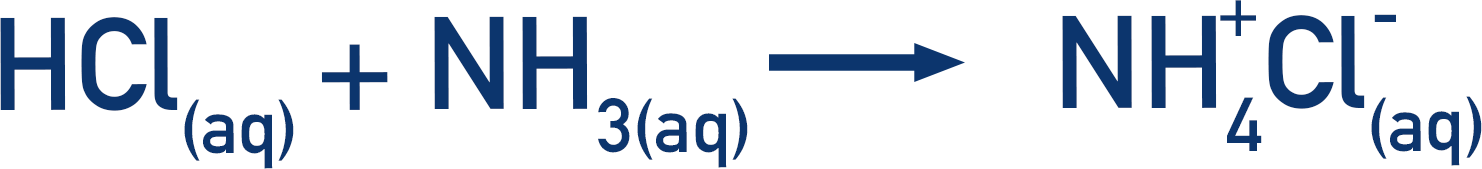Here the HCl has ‘given’ its proton to the ammonia, leaving behind a negatively charged chloride ion (Cl-). The ammonia has gained a proton and become a positively charged ammonium ion (NH+4). Chloride ions and ammonium ions are attracted to each other ionically, so ammonium chloride is formed.The HCl acts as an acid (giving away a proton) and the NH3 acts as a base (accepting the proton).

This seems straightforward and easy to follow, but the actual process that happens is a little more involved.

Remember everything here is aqueous (in water). Acids actually ‘give’ their protons to water molecules, not directly to a base. This forms hydroxonium ions (H3O+) and it is these ions that then go on to react with bases.

Let’s take hydrochloric acid and sodium hydroxide: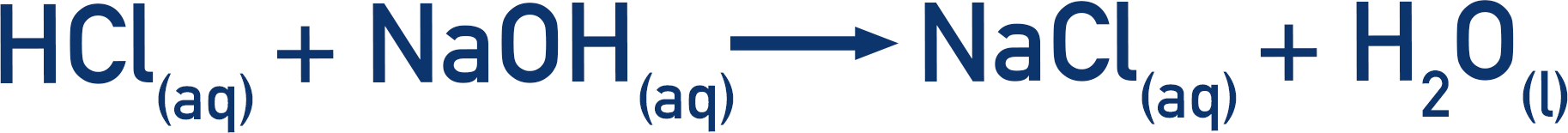Remember the acid is actually giving its proton to a water molecule to form a hydroxonium ion.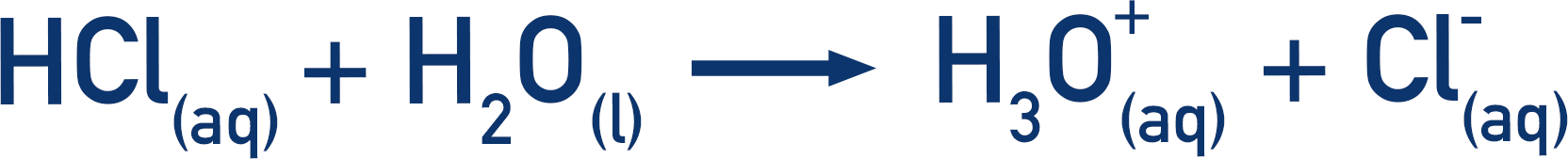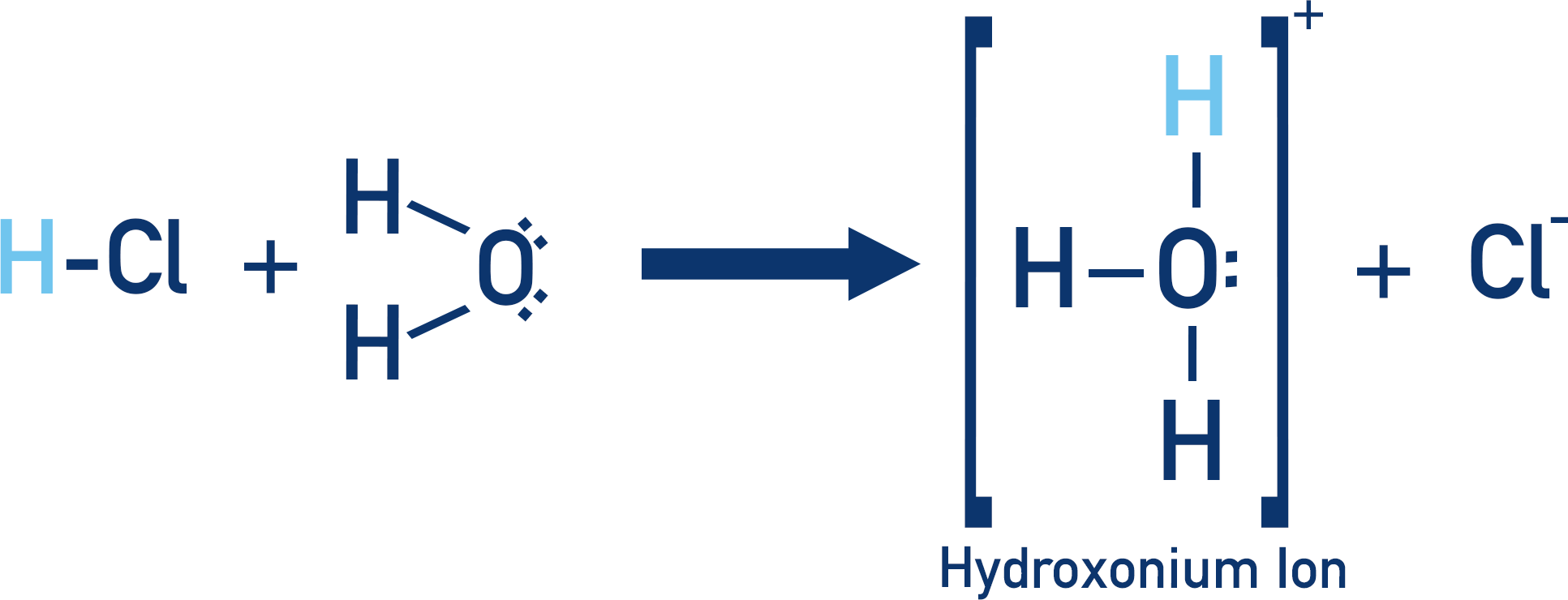This hydroxonium ion then reacts with the hydroxide ion (OH-) in the solution from the sodium hydroxide (NaOH) to form water.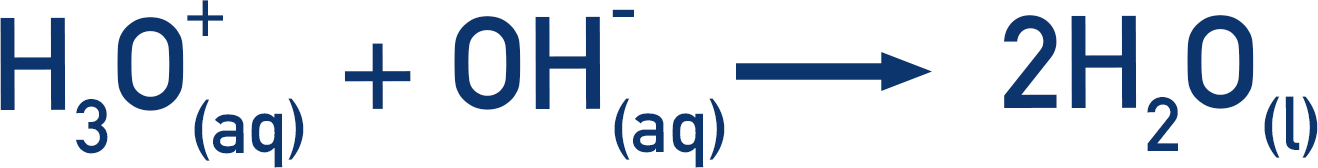If we combine these equations together, the water that reacts to form the hydroxonium ion reforms at the end, so it can be removed from the equation and we are left with:## Conjugate Acids and Conjugate Bases

As defined above, the Bronsted-Lowry theory proposes that acids donate protons (H+ ions) and bases accept protons.

In a reaction, when an acid dissociates it forms a negative ion. If this negative ion was to accept a proton, it would re-form the original acid and therefore would act as a base. Equally, if a base accepts a proton it could then later react and release the proton, acting as an acid!

We call these conjugate pairs.

An acid dissociates, forming its conjugate base. In reverse, a base can accept a proton (H+ ion) and form its conjugate acid.